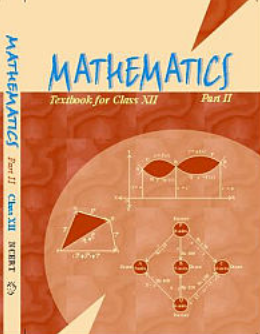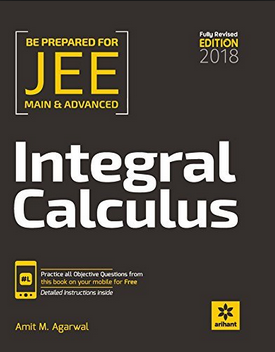# Differential equations (Weightage 3%)   Share

### Topics from Differential equations

• Formation of differential equations (5 concepts)
• Ordinary differential equations, their order and degree (8 concepts)
• Solution of differential equations by the method of separation of variables (12 concepts)
• Solution of homogeneous and linear differential equations (26 concepts)

### Important Books for Differential equations

••Exams
Articles
Questions